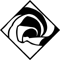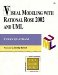# Complexity and Sequence Diagrams

 I l @ ve RuBoard

 Every time i teach a class, the question "How complex can a sequence diagram be?" is always asked. The answer I always give is "Keep them simple." The beauty of these diagrams is their simplicityit is very easy to see the objects, the object interactions, the messages between the objects, and the functionality captured by the scenario. The next question is usually "What do I do about conditional logic?" (all the if, then, else logic that exists in the real world). Here, you again have a very subjective answer. If the logic is simple, involving only a few messages, I usually add the logic to one diagram and use notes and scripts to communicate the choices to be made. On the other hand, if the if, then, else logic involves many complicated messages, I typically draw a separate diagramone for the if case, one for the then case, and one for the else case. This is done to keep the diagrams simple. If you desire , diagrams may be linked to one another. This allows the user to navigate through a set of diagrams. LINKING DIAGRAMS IN RATIONAL ROSESelect the Note icon from the toolbar. Click on the diagram to place the note. Select the diagram that you wish to link in the browser and drag the diagram onto the note. To navigate to the linked diagram, double-click on the note.
 I l @ ve RuBoardVisual Modeling with Rational Rose 2002 and UML (3rd Edition)
ISBN: 0201729326
EAN: 2147483647
Year: 2002
Pages: 134

Similar book on Amazon